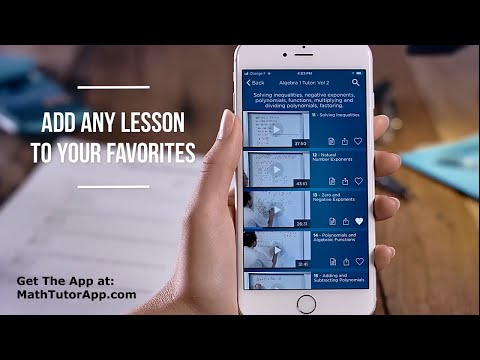# Math & Science Tutor - Algebra, Calculus, PhysicsEveryone
671
Offers in-app purchases1500+ Math Tutor Video Lessons in Basic Math, Algebra, Calculus, Physics, Chemistry, Engineering, Statistics. 500+ hours of step-by-step instruction.

Learn fast and get help in any subject by solving example problems step-by-step. Every lesson teaches the student how to solve problems, gain practice, and perform the calculations to score higher on exams and quizzes. All classes are taught assuming that the student has no knowledge of the subject.

Whether learning basic math, algebra, calculus, or advanced courses such as electrical engineering or mechanical engineering, this method is the fastest way to truly master the material.

Included Courses:

Basic Math (Arithmetic):
Addition, Subtraction, Multiplication, Division, Fractions, Ratio, Proportion, Percents, Word Problems.

- Algebra 1 and Algebra 2:
Real Numbers, Integers, Rational Numbers, Algebraic Fractions, Simplifying Expressions, Solving Equations, Multi Step Equations, Graphing, Quadratic Functions.

- Geometry
Lines, Rays, Planes, Quadrilaterals, Surface Area, Volume, Prisms, Parallel Lines, Geometric Theorems, Proofs, Circles, Circumference.

- College Algebra
Rational Functions, Shifting Functions, Sequences, Series, Matrix Algebra, Summation.

- Trigonometry & PreCalculus
Imaginary Numbers, Complex Numbers, Unit Circle, Sin, Cos, Tan, Trig Identities, Exponential Functions, Logarithmic Functions, Trigonometric Equations.

- Calculus 1
Limits, Derivatives, Integrals, Techniques of Integration, Substitution, Improper Integrals, Curve Sketching

- Calculus 2
Integration by Parts, Integration by Trig Substitution, Sequences, Series, Convergence, Implicit Differentiation

Calculus 3
Partial Derivatives, Line Integrals, Surface Integrals, Directional Derivatives, Green's Theorem, Stokes Theorem

- Differential Equations
Solving Differential Equations, Graphing Solutions, Systems of Equations

- Calculator Tutorials
Texas Instruments TI-84, TI-89 Graphing Calculator Tutorial

- Physics 1
Motion, Projectile Motion, Torque, Momentum, Work, Energy, Friction, Fluids, Pressure

Physics 2
Temperature, Heat, Thermodynamics, Waves, Simple Harmonic Motion

Physics 3
Electricity, Magnetism, Maxwell's Equations, Electric Field, Magnetic Field

- Chemistry
Atoms, Compounds, Chemical Reactions, Stoichiometry, Gas Laws, Redox Reactions

- Probability & Statistics
Sampling Statistics, Central Limit Theorem, Hypothesis Testing, Linear Regression, Correlation, ANOVA

- Electrical Engineering
Circuit Analysis, Node Voltage, Mesh Current, Dependent Sources, Thevenin Circuits, Phasors, 3 Phase Circuits

- Mechanical Engineering
Statics, Vector Mechanics, Equilibrium, Forces

- Engineering Math
Linear Algebra, Laplace Transform, Matrices

- Java Programming
Objects, Classes, For Loops, While Loops, Variables, Methods

- Matlab, MS Word, MS Excel

- Science Experiments

App Features:
- Mark favorite lessons for later viewing.
- Recently watched videos list.
- Search all lessons for any topic.
- View featured courses.
- View recently released courses.
- Worksheets for selected courses.
- Share lessons via email & social media.

Excel in school. Learn any subject fast by solving problems step-by-step. Our lessons have helped thousands of students achieve success!

- Most lessons in the app are free. For \$19.99 a month, you will get access to all 1,500+ lessons and courses.
- Your subscription will automatically renew at \$19.99 each month, billed through your account.
- You can cancel anytime by turning off auto-renew in your account settings.
- The subscription automatically renews every month unless auto-renew is turned off at least 24 hours before the end of the current period.
- No cancellation of the current subscription is allowed during the active subscription period.

Collapse

Review Policy
4.5
671 total
5
4
3
2
1

## What's New

New lessons in Algebra, Calculus, and Electrical Engineering.
Collapse

Updated
May 22, 2018
Size
9.0M
Installs
100,000+
Current Version
1.0.9
Requires Android
4.4 and up
Content Rating
Everyone
In-app Products
\$19.99 per item
Permissions
Offered By
Math Tutor DVD, LLC
Developer